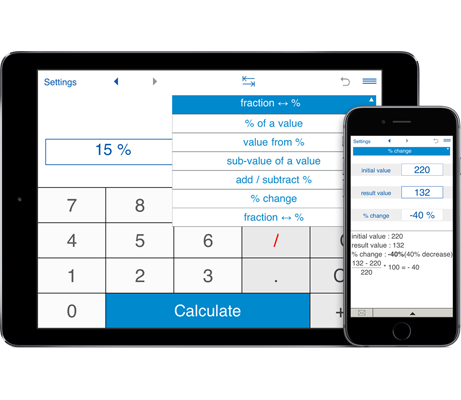# Percentage Calculator

Try a comprehensive must-have app that helps you with various percentage calculations! You can add and subtract percentages, calculate VAT, discounts, percentage change, tips, sales price, percentage off, convert between fractions and percents and more. While providing powerful built-in solutions and expanded preference settings, it is easy to use and perfect for both simple and complex calculations.

## Calculate percentages, VAT and discounts on the fly

Percentage Calculator from Intemodino is a universal solution for all your percentage related problems:
- Find a given percent of a number - what is X% of Y?
- Find the whole number if you know that X if Y% of the whole number?
- Calculate what percent of X is Y.
- Add or subtract a percent to/from a number.
- Calculate percentage increase or decrease.
- Calculate percentage difference.
- Fractions to percents and percents to fractions conversion.
- Calculate price with or without VAT, VAT amount and VAT rate.
- Calculate the sale price, discounted price, discounts and percentage of savings.
- View calculations and share them via email.

### How to use Percentage Calculator

While percentage calculations are used in a wide variety of fields, they can be difficult to perform by hand. Intemodino Percentage Calculator handles practically any percentage problem and can be used to save time and accurately perform daily calculations with percents.

#### How to determine the percentage of a number

Example: what is 5% of 20?
To find the percentage of a value, choose "Percentages" and then "% of a value" from the drop-down menu. Now enter 5 in the frist field, 20 in the second field and click Calculate.

#### Find the whole number when you know the percentage and the sub-value

Example: 4 is 30% of what?
Choose "value from %", input 4 and 30 in the corresponding fields and press Calculate. The calculator also shows the detailed explanation.

#### How to calculate the percentage of one number vs. another number

Example: 20 is what percent of 150?
If you want to find what percent of Y is X, choose "sub-value of a value", input both values and press Calculate. The app shows both the result and the solution.

#### How to add or subtract a percentage

Need to find out how much larger or smaller an initial number would be when increased or decreased by a given percent? Just choose "Percentages" in the first screen, choose "add / subtract %" from the drop-down menu, enter the initial number and the percentage, choose the desired operation and click "Calculate".
Negative values and percentages are possible. Simply tap the "+/-" button to put a minus sign.

#### How to calculate the percentage change

To find the percentage increase or decrease between two numbers, choose "percentage change" and enter the initial and result values. The app supports both positive and negative values.

#### How to convert a fraction to percent and vice versa

To solve fraction to percentage and percentage to fraction conversions, select "% to /from fraction", input a fraction that you would like to convert to a percent value and press Calculate. To convert the percent value into a fraction, press the left right arrows button in the top menu and enter the percent value.